Understanding multivariate statistics requires mastery of high-dimensional geometry and concepts in linear algebra such as matrix factorizations, basis vectors, and linear subspaces. Graphs can help to summarize what a multivariate analysis is telling us about the data. This article looks at four graphs that are often part of a principal component analysis of multivariate data. The four plots are the scree plot, the profile plot, the score plot, and the pattern plot.

The graphs are shown for a principal component analysis of the 150 flowers in the Fisher iris data set. In SAS, you can create the graphs by using PROC PRINCOMP. By default, the scatter plots that display markers also label the markers by using an ID variable (such as name, state, patient ID, ...) or by using the observation number if you do not specify an ID variable. To suppress the labels, you can create an ID variable that contains a blank string, as follows:

```data iris; set Sashelp.iris; id = " "; /* create empty label variable */ run;```

The following statements create the four plots as part of a principal component analysis. The data are the measurements (in millimeters) of length and width of the sepal and petal for 150 iris flowers:

```ods graphics on; proc princomp data=iris /* use N= option to specify number of PCs */ STD /* optional: stdize PC scores to unit variance */ out=PCOut /* only needed to demonstate corr(PC, orig vars) */ plots=(scree profile pattern score); var SepalLength SepalWidth PetalLength PetalWidth; /* or use _NUMERIC_ */ ID id; /* use blank ID to avoid labeling by obs number */ ods output Eigenvectors=EV; /* to create loadings plot, output this table */ run;```

The principal components are linear combinations of the original data variables. Before we discuss the graph, let's identify the principal components and interpret their relationship to the original variables. The linear coefficients for the PCs (sometimes called the "loadings") are shown in the columns of the Eigenvectors table.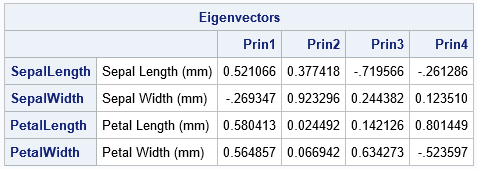• The first PC is the linear combination PC1 = 0.52*SepalLength – 0.27*SepalWidth + 0.58*PetalLength + 0.56*PetalWidth. You can interpret this as a contrast between the SepalWidth variable and an equally weighted sum of the other variables.
• For the second PC, the coefficients for the PetalLength and PetalWidth variables are very small. Therefore, the second PC is approximately PC2 ≈ 0.38*SepalLength + 0.92*SepalWidth. You can interpret this weighted sum as a vector that points mostly in the direction of the SepalWidth variable but has a small component in the direction of the SepalLength variable.
• In a similar way, the third PC is primarily a weighted contrast between the SepalLength and PetalWidth variables, with smaller contributions from the other variables.
• The fourth PC is a weighted contrast between the SepalWidth and PetalLength variables (with positive coefficients) and the SepalLength and PetalWidth variables (with negative coefficients).

Note that the principal components (which are based on eigenvectors of the correlation matrix) are not unique. If v is a PC vector, then so is -v. If you compare PCs from two different software packages, you might notice that a PC from one package is the negative of the same PC from another package.

### The scree plot

Recall that the main idea behind principal component analysis (PCA) is that most of the variance in high-dimensional data can be captured in a lower-dimensional subspace that is spanned by the first few principal components. You can therefore to "reduce the dimension" by choosing a small number of principal components to retain. But how many PCs should you retain? The scree plot is a line plot of the eigenvalues of the correlation matrix, ordered from largest to smallest. (If you use the COV option, it is a plot of the eigenvalues of the covariance matrix.)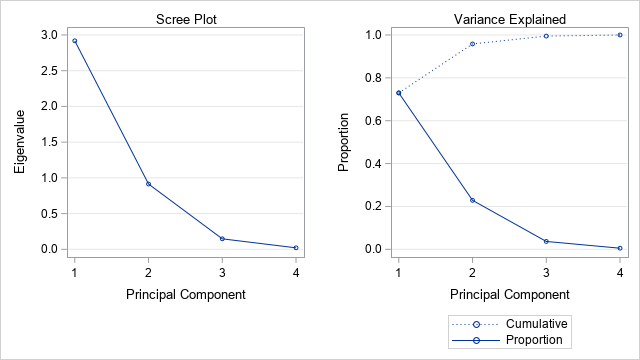You can use the scree plot as a graphical tool to help you choose how many PCs to retain. In the scree plot for the iris data, you can see (on the "Variance Explained" plot) that the first two eigenvalues explain about 96% of the variance in the four-dimensional data. This suggests that you should retain the first two PCs, and that a projection of the data onto the first to PCs will give you a good way to visualize the data in a low-dimensional linear subspace.

### The profile plot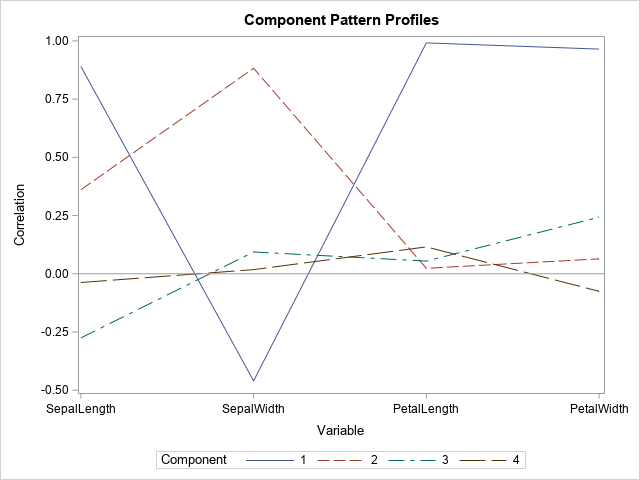The profile plot shows the correlations between each PC and the original variables. To some extent, you can guess the sign and the approximate magnitude of the correlations by looking at the coefficients that define each PC as a linear combination of the original variables. The correlations are shown in the following "Component Pattern Profiles" plot.

The profile plot reveals the following facts about the PCs:

• The first PC (solid blue line) is strongly positively correlated with SepalLength, PetalLength, and PetalWidth. It is moderately negatively correlated with SepalWidth.
• The second PC (dashed reddish line) is positively correlated with SepalLength and SepalWidth.
• The third and fourth PCs have only small correlations with the original variables.

The component pattern plot shows all pairwise correlations at a glance. However, if you are analyzing many variables (say 10 or more), the plot will become very crowded and hard to read. In that situation, the pattern plots are easier to read, as shown in the next section.

### The pattern plots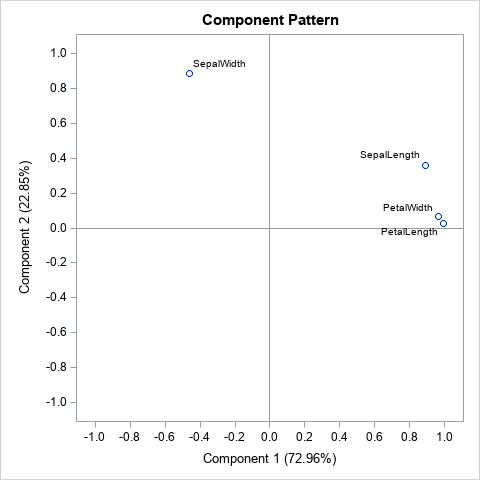The output from PROC PRINCOMP includes six "component pattern" plots, which show the correlations between the principal components and the original variables. Because there are four PCs, a component pattern plot is created for each pairwise combination of PCs: (PC1, PC2), (PC1, PC3), (PC1, PC4), (PC2, PC3), (PC2, PC4), and (PC3, PC4). In general, if there are k principal components, there are N(N-1)/2 pairwise combinations of PCs.

Each plot shows the correlations between the original variables and the PCs. For example, the graph shown to the right shows the correlations between the original variables and the first two PCs. Each point represents the correlations between an original variable and two PCs. The correlations with the first PC are plotted on the horizontal axis; the correlations with the second PC are plotted on the vertical axis.

Six graphs require a lot of space for what is essentially a grid of sixteen numbers. If you want to see the actual correlations in a table, you can call PROC CORR on the OUT= data set, as follows:

```/* what are the correlations between PCs and orig vars? */ proc corr data=PCOUT noprob nosimple; var SepalLength SepalWidth PetalLength PetalWidth; with Prin1-Prin4; run;```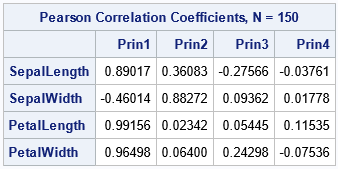If you know you want to keep only two PCs, you can use the N=2 option on the PROC PRINCOMP statement, which will reduce the number of graphs that are created.

### The score plots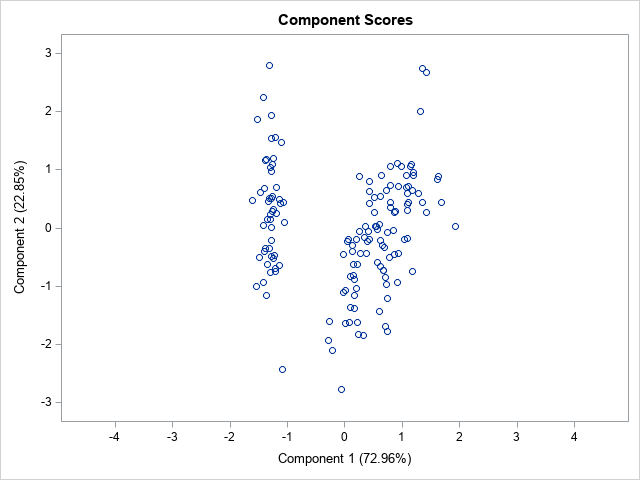The score plots indicate the projection of the data onto the span of the principal components. As in the previous section, this four-dimensional example results in six score plots, one for each pairwise combination of PCs. You will get different plots if you create PCs for the covariance matrix (the COV option) as opposed to the correlation matrix (the default). Similarly, if you standardize the PCs (the STD option) or do not standardize them (the default), the corresponding score plot will be different.

The score plot for the first two PCs is shown. Notice that it uses equal scales for the axes. The graph shows that the first principal component separates the data into two clusters. The left cluster contains the flower from the Iris setosa species. You can see a few outliers, such as one setosa flower whose second PC score (about -2.5) is much smaller than the other setosa flowers.

ODS graphics provide an easy way to generate a quick look at the data. However, if you want to more control over the graphs, it is often simplest to output the results to a SAS data set and customize the plots by hand. You can use the OUT= option to write the PCs to a data set. The following call to PROC SGPLOT creates the same score plot but colors the markers by the Species variable and adds a grid of reference lines.

```title "Score Plot"; title2 "Observations Projected onto PC1 and PC2"; proc sgplot data=PCOut aspect=1; scatter x=Prin1 y=Prin2 / group=species; xaxis grid label="Component 1 (72.96%)"; yaxis grid label="Component 2 (22.85%)"; run;```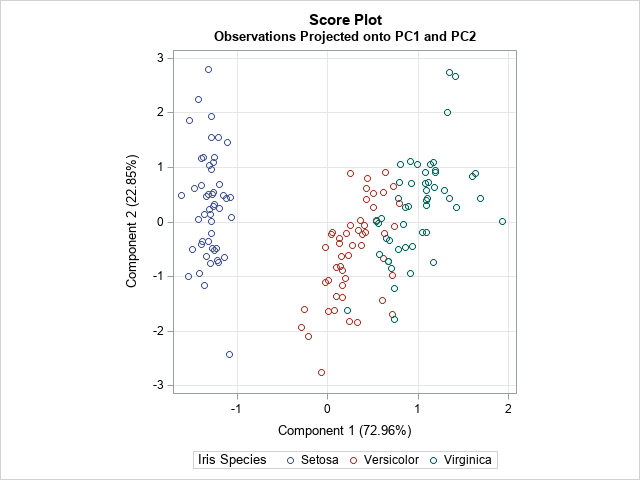A loadings plot is a plot of two columns of the Eigenvectors table. PROC PRINCOMP does not create a loadings plot automatically, but there are two ways to create it. One way is to use the ODS OUTPUT to write the Eigenvectors table to a SAS data set. The previous call to PROC PRINCOMP created a data set named EV. The following call to PROC SGPLOT creates a score plot that projects the observations onto the first two PCs.

```title "Loadings Plot"; title2 "Variables Projected onto PC1 and PC2"; proc sgplot data=EV aspect=1; vector x=Prin1 y=Prin2 / datalabel=Variable; xaxis grid label="Component 1 (72.96%)"; yaxis grid label="Component 2 (22.85%)"; run;```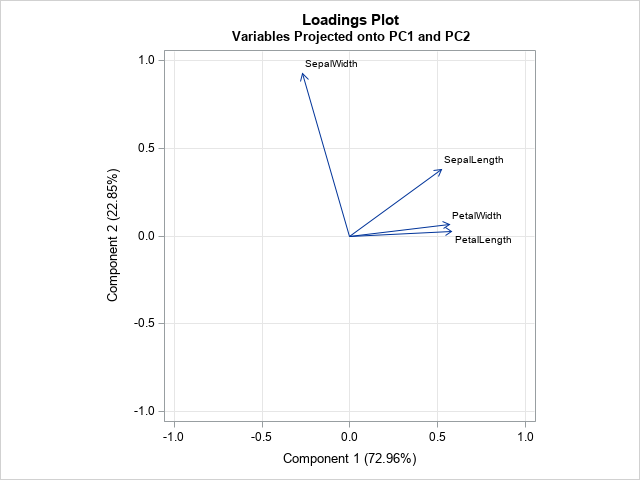The loadings plot shows the relationship between the PCs and the original variables. You can use the graph to show how the original variables relate to the PCs, or the other way around. For example, the graph indicates that the PetalWidth and PetalLength variables point in the same direction as PC1. The graph also shows that the second PC is primarily in the direction of the SepalWidth variable, with a small shift towards the direction of the SepalLength variable. The second PC is essentially orthogonal to the and PetalWidth and PetalLength variables.

The second way to create a loadings plot is to use PROC FACTOR, as shown by the following statements. To documentation for PROC FACTOR compares the PROC FACTOR analysis to the PROC PRINCOMP analysis.

```proc factor data=iris N=2 /* use N= option to specify number of PCs */ method=principal plots=(initloadings(vector)); var SepalLength SepalWidth PetalLength PetalWidth; /* or use _NUMERIC_ */ run;```

### Summary

In summary, PROC PRINCOMP can compute a lot of graphs that are associated with a principal component analysis. This article shows how to interpret the most-used graphs. The scree plot is useful for determining the number of PCs to keep. The component pattern plot shows the correlations between the PCs and the original variables. The component pattern plots show similar information, but each plot displays the correlations between the original variables and a pair of PCs. The score plots project the observations onto a pair of PCs. The loadings plot project the original variables onto a pair of PCs.

When you analyze many variables, the number of graphs can be overwhelming. I suggest that you use the WHERE option in the ODS SELECT statement to restrict the number of pattern plots and score plots. For example, the following statement creates only two pattern plots and two score plots:

``` ods select Eigenvectors ScreePlot PatternProfilePlot where=(_label_ in: ('2 by 1','4 by 3')); /* limit pattern plots and score plots */```

There is one more plot that is sometimes used. It is called a "biplot" and it combines the information in a score plot and a loadings plot. I will discuss the biplot in a subsequent article.

The post How to interpret graphs in a principal component analysis appeared first on The DO Loop.